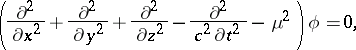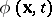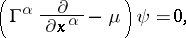# Is there any chance a Higgs boson is always decohered even when traveling in an unobserved matter wave?

#### pittsburghjoe

How sure are we that an excitation in the Higgs field is a quantum wave?

#### sarajo

im not sure at all

#### Hartmann352

There is no Higgs wave , as the Higgs boson has a mass, in contrast to the photon and the graviton (if it exists). The Higgs boson is an excitation of the Higgs field, and its discovery is important because it validated the standard model of particle physics, which needs the Higgs field for the generation of masses.

A Higgs boson, if it were long lived, would have left a point by its decay in a detector, and an accumulation of such points would show a wave nature, given similar boundary conditions as in the double slit experiments.

An electron beam can show collectively the patterns, thus in principle able to transfer energy in a medium, with wave properties, but this wave cannot be confused with the theoretical waves built up by zero mass gauge bosons macroscopically that do not need a medium to appear and transfer energy in spacetime.

Taking the Higgs boson (which is not exactly the same as the Higgs field), we have an unstable particle. Once created, it decays very quickly to other particles. So, it is very difficult to observe such wave-like phenomena with the Higgs boson. However, being a massive scalar field, it obeys the Klein-Gordon equation, which is a wave equation. So, in principle, it can act as a wave, if only it were not so unstable.

The subsequent application of the Klein–Gordon equation as a relativistic quantum equation proved possible in quantum field theory but not in quantum mechanics. An interpretation of the Klein–Gordon equation was given as an equation for fields of particles of zero spin. The Klein–Gordon equation is applied in the description of-mesons and corresponding fields; it plays the role of one of the fundamental equations of quantum field theory.

The Klein–Gordon equation is a linear homogeneous second-order partial differential equation with constant coefficients:(1)
whereis a (pseudo-) scalar function, in the general case — complex,andis the rest mass of the particle. Ifis a real function, then the Klein–Gordon equation describes neutral (pseudo-) scalar particles, while whenis complex it describes charged particles.
In the latter case equation (1) is supplemented by the equation for the complex-conjugate scalar function:(2)

As for all relativistic equations, the Klein–Gordon equation can be expressed in the form of the Dirac equation, that is, it can be reduced to a first-order linear equation:(3)

Last edited:
•pittsburghjoe

#### pittsburghjoe

You are assuming anything with mass is always physical?